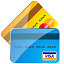Mod 10 (Luhn Algorithm) Checksum Validator

QHow to validate Mod 10 (Luhn Algorithm) checksum?

In order to help your programming or testing tasks, FYIcenter.com has designed this online tool for you to validate the Mod 10 (Luhn Algorithm) checksum digit of any given credit card, ATM card, or SIM card number.

All you need to do is to enter the card number, and click the "Validate" button. Validation result will be displayed in the result area.

Card Number:

✍: FYIcenter.com

A

Validation result by FYIcenter.com - Card Number:

Specified Card Number: Valid

```Card Number: 4417123456789113
Provided checksum: 3
Expected checksum: 3
```

What is the Mod 10 checksum?

Mod 10 checksum is the last digit of your credit card, ATM card or many other financial card numbers. It is calculated based on the other digits of the number using Mod 10, also called Luhn algorithm.

The main purpose of the checksum digit in a multi-digit number is to allow the user interface to detect mistyped digits quickly. For example, 4417123456789113 is a credit card number with 3 as the valid Mod 10 checksum digit at the end. If someone mistypes 0 instead of 9 and enters 4417123456780113 as the credit card number. The user interface will detect that 3 is no longer a valid Mod 10 checksum and reject this credit card number.

Here is how Mod 10 (Luhn algorithm) checksum validation works:

• Remove the checksum digit, which is the last digit of the number.
• Reverse positions of all remaining digits.
• For every digit in an odd position, double its value and subtract 9 if result is over 9.
• Sum up values from all digits.
• Add the checksum digit to the sum.
• If the sum is divisible by 10 (mod 10), then the checksum is valid.

For example, given credit card number "4417123456789113":

• Remove the checksum digit "3": "441712345678911".
• Reverse positions: "1198 7654 3217 144".
• Double values on odd positions and subtract 9 if needed: "219856146227248".
• Sum up all digits: 77.
• Add the checksum digit: 80.
• Mod 10: 0. So the checksum is valid.

2022-08-05, 4988👍, 1💬